# Trimesh¶

Title
TriMesh Element
Dependencies
Bokeh
Backends
Matplotlib
Bokeh
In :
import numpy as np
import holoviews as hv

from holoviews import opts
from scipy.spatial import Delaunay

hv.extension('matplotlib')A TriMesh represents a mesh of triangles represented as the simplexes and vertexes. The simplexes represent the indices into the vertex data, made up of three indices per triangle. The mesh therefore follows a datastructure very similar to a graph, with the abstract connectivity between nodes stored on the TriMesh element itself, the node or vertex positions stored on a Nodes element and the concrete EdgePaths making up each triangle generated when required by accessing the edgepaths attribute.

Unlike a Graph each simplex is represented as the node indices of the three corners of each triangle rather than the usual source and target node.

We will begin with a simple random mesh, generated by sampling some random integers and then applying Delaunay triangulation, which is available in SciPy. We can then construct the TriMesh by passing it the simplexes and the vertices (or nodes).

In :
n_verts = 100
pts = np.random.randint(1, n_verts, (n_verts, 2))
tris = Delaunay(pts)

trimesh = hv.TriMesh((tris.simplices, pts))
trimesh

Out: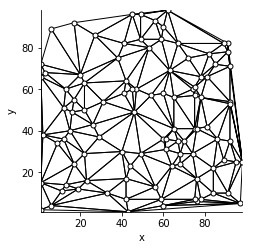To make this easier TriMesh also provides a convenient from_vertices method, which will apply the Delaunay triangulation and construct the TriMesh for us:

In :
hv.TriMesh.from_vertices(np.random.randn(100, 2))

Out: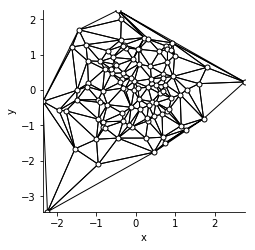Just like the Graph element we can access the Nodes and EdgePaths via the .nodes and .edgepaths attributes respectively.

In :
trimesh.nodes + trimesh.edgepaths

Out: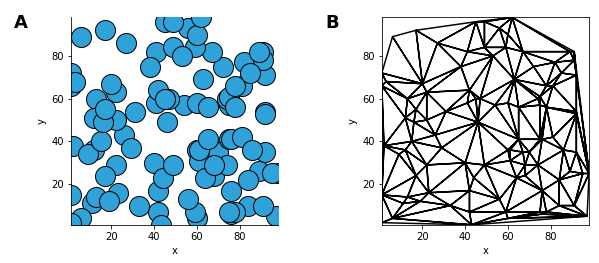Now let's make a slightly more interesting example by generating a more complex geometry. Here we will compute a geometry, then apply Delaunay triangulation again and finally apply a mask to drop nodes in the center.

In :
# First create the x and y coordinates of the points.
n_angles = 36

angles = np.linspace(0, 2*np.pi, n_angles, endpoint=False)
angles = np.repeat(angles[..., np.newaxis], n_radii, axis=1)
angles[:, 1::2] += np.pi/n_angles

nodes = np.column_stack([x, y, z])

# Apply Delaunay triangulation
delauney = Delaunay(np.column_stack([x, y]))

xmid = x[delauney.simplices].mean(axis=1)
ymid = y[delauney.simplices].mean(axis=1)


Once again we can simply supply the simplices and nodes to the TriMesh.

In :
hv.TriMesh((simplices, nodes))

Out: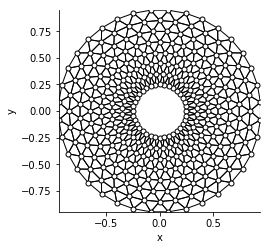We can also do something more interesting, e.g. by adding a value dimension to the vertices and coloring the edges by the vertex averaged value using the edge_color plot option:

In :
hv.TriMesh((simplices, hv.Points(nodes, vdims='z'))).opts(
opts.TriMesh(cmap='viridis', edge_color='z', filled=True, fig_size=200))

Out: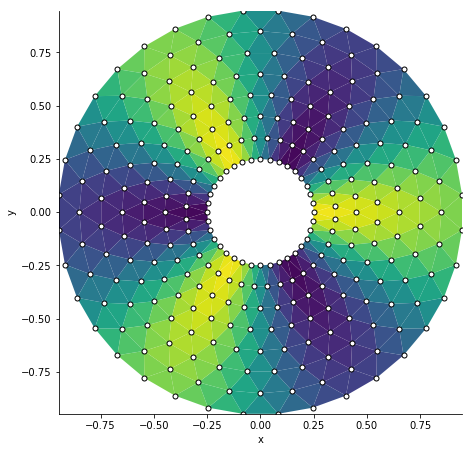For full documentation and the available style and plot options, use hv.help(hv.TriMesh).